# The Haybaler Problem: Several Ways to Solve

We often compare math problems to puzzles; and some puzzles are math problems. I want to devote a couple posts to interesting puzzles that can be attacked in various ways. Here, we are given the weights of every possible pair of hay bales, and have to work out the individual weights. This classic can be solved with lots of algebra, or a little carefully managed algebra, or just by logical reasoning.

## Solving by a system of equations

We’ve see this problem with a couple different sets of numbers; here is the first version we got, in 1996:

Weighing Bales of Hay

You have 5 bales of hay. Instead of being weighed individually, they were weighed in all possible combinations of two: bales 1 and 2, 1 and 3, 1 and 4, 1 and 5, bales 2 and 3, and bales 2 and  4 etc. The weights of each of these combinations were written down and arranged in numeric order, without keeping track of which weight matched which pair of bales. The weights in kilograms are 110, 112, 113, 114, 115, 116, 117, 118, 120, and 121.

How much does each bale weigh? Is there more than one possible set of weights?  Explain your answer.

Note that with 5 bales, there are $${5\choose 2} = 10$$ possible pairs, so we do have all pairs, none of which are equal.

Doctor Alain showed how to start solving the problem, leaving it unfinished:

Let the 5 bales be in increasing weight order and call their weights B1, B2, B3, B4 and B5. There can't be 2 bales of the same weight or else there would be pairs of bales with the same weight (if B1 = B2 then B1 + B3 = B2 + B3). The lightest pair is 110 kg so

(i) B1 + B2 = 110.

The second lightest pair is 112 kg so

(ii) B1 + B3 = 112.

The heaviest pair is 121 kg so

(iii) B4 + B5 = 121.

The second heaviest pair is 120 kg so

(iv) B3 + B5 = 120.

From (iv) and (ii) we get B5 = B1 + 8. If we put this back in (iii) we get

(v) B1 + B4 = 113.

so the third lightest pair is bale 1 and bale 4. The fourth lightest pair can be bales 1 and 5 OR bales 2 and 3.

So (vi.a) B1 + B5 = 114    OR    (vi.b) B2 + B3 = 114.

Try each of these equations. One works out fine - the other doesn't.

With ten numbers, we could have written ten equations if we knew which sum is which number; we only need five equations to solve for five variables. That’s the problem. We have started with four equations, based on the largest and smallest numbers; the fifth, being derived from those, adds no information to the system of equations, but does help decide what the other sums have to be. The first four, plus one of the two options for the third, will yield our solution.

Not a bad method, though you might prefer to avoid the need to try two possibilities.

For a different method with similar difficulty (and with different numbers), see this answer by Doctor Pete in 1997:

Weighing Bales of Hay

### Algebra and elimination

Earlier in 1997, we had a similar question (with the same smaller numbers):

Weight of Each Bale of Hay

You have a question like the one I am going to ask but with different numbers, so if you could help me ...

You have 5 bales of hay. Instead of being weighed individually, they are weighed in all possible combinations of two: bales 1 and 2, 1 and 3, 1 and 4, 1 and 5, bales 2 and 3, and bales 2 and 4, etc.

The weight of each of these combinations is written down and the weights are arranged in numeric order, without keeping track of which weight matches which pair of bales.

The weights in kilograms are 80, 82, 83, 84, 85, 86, 87, 88, 90, and 91.

How much does each bale weigh? Is there more than one possible set of weights?  Explain your answer.

Doctor Rob chose to use a very different method with no explicit algebra:

First, notice that no two bales can have the same weight, since not enough of the numbers appear twice (in fact, no number appears twice).  If two bales were of equal weight we should see at least three pairs of equal numbers.

Second, notice that if you add up all the numbers and divide by four, you will get the total weight of the five bales, 214 kilograms.

Each of the five weights has been added to each of the other 4, so each appears 4 times among the 10 sums. Adding the 10 sums, we get the sum of 4 times each weight. If the weights are A, B, C, D, and E, we now know that $$A+B+C+D+E = 214$$.

Third, notice that the largest number is the weight of the two heaviest bales, and the smallest number is the weight of the two lightest bales.

Algebraically, $$A+B = 80$$ and $$D+E = 91$$.

Now if you subtract the largest and the smallest numbers from the total weight, you will get the weight of the middle bale, 43 kilograms. Since 43 added to each of the other four weights are integers, all the weights must be integers.

Adding $$A+B = 80$$ and $$D+E = 91$$, we get $$A+B+D+E = 171$$, and subtracting this from $$A+B+C+D+E = 214$$, we get $$C = 43$$. And this tells us that A and B must be less than 43, while D and E must be greater than 43.

(You may not have noticed that we aren’t told that the weights are all integers or not; it is natural to assume that, but it might not have been so! Now we are sure.)

Now we leave the pieces that can be expressed algebraically, and start using the fact that the weights are distinct integers:

Then the lightest two bales must have unequal weights at most 42, and which sum to 80, so they are either (38,42) or (39,41). Since the number 81 is missing, no bale can have weight 81 - 43 = 38, so (38,42) is eliminated. Thus the three lightest bales have weights 39, 41, and 43. This accounts for the numbers 80, 82, and 84. The two heaviest bales must have unequal weights that sum to 91 and which are at least 44, so they are (44,47) or (45,46). Since the number 89 is missing, no bale can have weight 89 - 43 = 46, so the last two weights are 44 and 47.

Since $$A+B = 80$$ and neither number can be more than 42, they must be either $$38+42 = 80$$ or $$39+41 = 80$$. But if A were 38, then another of the sums would be $$38+43 = 81$$, and that can’t happen. So we’ve eliminated one pair, and we know that $$A = 39$$ and $$B = 41$$. Similarly we find that $$D = 44$$ and $$E = 47$$.

So the weights are  39, 41, 43, 44, and 47 kg.

Now check:

39 + 41 = 80
39 + 43 = 82
39 + 44 = 83
39 + 47 = 86
41 + 43 = 84
41 + 44 = 85
41 + 47 = 88
43 + 44 = 87
43 + 47 = 90
44 + 47 = 91

The check is required, because until now we haven’t used all of the sums. But it works.

Some people would complain that this is not as “mathematical” as one of the algebraic methods, but that is not true; much of mathematics is more logical than algebraic.

## An algebraic shortcut

In 2002 we again got essentially the same question:

The Haybaler Problem: Reflections and Refinements

Say you have five bales of hay.

For some reason, instead of being weighed individually, they were weighed in all possible combinations of two: bales 1 and 2, bales 1 and 3, bales 1 and 4, bales 1 and 5, bales 2 and 3, etc.

The weights of each of these combinations were written down, and arranged in numerical order, WITHOUT KEEPING TRACK OF WHICH WEIGHT MATCHED WHICH PAIR OF BALES.

The weights in kilograms are 80, 82, 83, 84, 85, 86, 87, 88, 90, and 91.

How much does each bale weight?

Is there more than one set of possible weights? Explain how you know.

Once your are done looking for solutions, look back over the problem to see if you can find some easier or more efficient way to find the weights.

(Note that last paragraph: That is Polya’s final step, and is good teaching!)

This time Doctor Greenie answered, giving a quick algebraic solution that has much in common with the elimination method. It happens that, because we already had several such answers in the archive, this answer was not published at the time; we’ll see why it was below. After first referring to the three answers we already had (in the order I have listed them), he offered his method:

Here is my personal approach to this problem, which is quite similar to the method used on the third page referenced above:

(1) Since the combined weights of the bales two at a time are all different, the individual weights of the bales are all different (if they were not, some of the weights obtained by weighing two bales at a time would be the same)

(2) Let the weights of the bales, from lightest to heaviest, be denoted by a, b, c, d, and e

This is how all answers have begun.  So is the next bit:

(3) We can logically conclude that

a + b = 80  [lightest combined weight = two lightest bales]
a + c = 82  [second lightest weight = lightest plus third lightest]
c + e = 90  [second heaviest weight = heaviest plus third heaviest]
d + e = 91  [heaviest combined weight = two heaviest bales]

(A common error in trying to solve this problem is to think that, for example, the third lightest combined weight is a + d; however, it could also be b + c. The four combinations above are the only ones we can logically be sure of.)

That is a valuable addition: in observing someone solve a problem, it is important not only to see what he does (here, identifying those four pairs), but also what he does not do! If you were to try solving without thinking very carefully, momentum might lead you to write more equations that are not necessarily true. Math requires this level of care.

(4) Now here is the key to my method: If you add up all the figures for the bales weighed two at a time, then each bale gets counted in that grand total 4 times -- once when weighed with each of the other bales. So you can find the sum of the weights of the 5 bales:

a + b + c + d + e = (80 + 82 + 83 + 84 + 85 + 86 + 87 + 88 + 90 + 91)/4

You will recognize this from Doctor Rob’s method, though he didn’t express it algebraically. This sum is 214.

He briefly describes how to finish up:

(5) Using two of the results from (3) above, we can find a + b + d + e; then, comparing that sum to the sum a + b + c + d + e which we know from (4), we can find the value of c

Once we have the value of c, the weights of the other bales are found very easily, using the equations in (3).

Let’s do that. We now know that $$c = 214 – (80 + 91) = 43$$. Putting that into the second equation, $$a + c = 82$$, we find that $$a = 82 – 43 = 39$$, and from the third equation, $$c + e = 90$$, we find that $$e = 90 – 43 = 47$$. Then from the first equation, $$a + b = 80$$, we get $$b = 80 – 39 = 41$$; and from the fourth equation, $$d + e = 91$$, we find that $$d = 91 – 47 = 44$$.

This is what I got as an answer: a = 39, b = 41, c = 43, d = 44, e = 47

Is that correct? Is that the only possible answer and why/why not?

The answer is correct, and either way of stating it (listing weights, or identifying individual bales in order) is good. Doctor Greenie acknowledged that:

Those are the numbers I got.

We know there is only one solution because there is nothing in our solution process where we had to make an arbitrary choice or make a guess as to where to go next. The equations we used exactly described the problem, and that set of equations had only one answer.

## Reducing the numbers

Then, 11 years later (2013) a reader (Jim) wrote us, having apparently read only the first answer above (1996), and suggesting a better way. I responded, pointing out that his answer was much like Doctor Rob’s and then quoting for him Doctor Greenie’s not-yet-published answer above, which is even more like it. As a result, the latter was then added to the archive, and Jim’s communication was appended to it – giving the accidental impression that it is a response to Doctor Greenie, when in fact it is responding to Doctor Alain. Here it is:

I'd like to explain a cleaner way to finish solving the problem, and provide generalizations that can help students solve other "equivalent" problems.

When I first saw the problem, I solved it as a discrete math problem, using very little (almost no) linear algebra. That is a bit tedious -- but it worked well for my daughter, who didn't have linear algebra skills.

The solution you gave starts with linear algebra, but then (in the last step) views the problem as a discrete math problem, asking you to "try" a limited set of possibilities. It turns out that you can finish the problem quite nicely with *only* linear equations, which also proves uniqueness.

The critical realization is that the sum of all the given pair-wise weights is exactly 4 times the sum of all the actual hay bale weights. As a result, is is easy to calculate what the sum of all the bale weights are. In linear algebra terms, "you now have a 5th equation" to accompany the first four (re: sum of the 1st and 2nd; sum of the 1st and 3rd; sum of the 4th and 5th, sum of the 3rd and 5th).

I’ll be showing my own “discrete math” (logical) method soon.

But there is a much nicer way to solve these -- a way that kids who haven't seen simultaneous equations can understand.

Since you realized that the least and second-to-least bales summed to one number, and the largest two bales summed to a second number, you can subtract that from the "sum of all 5 bales." This instantly gives you the weight of the middle bale. :-)

It is then easy to substitute back into the equations you had earlier and get all the answers.

As an additional benefit, this simplifies the problem, which -- in my opinion -- is always nice.

This summarizes Doctor Greenie’s method.

Now Jim added a new insight we haven’t seen yet:

I've seen a couple of variants of the hay baler problem on the Internet, but I didn't see any solutions that took advantage of the following insight.

When given some set of pairwise weight sums, you can generally reduce the problem by asking: What would happen if I took away the same weight from each hay bale?

For example, with sums of 110, 112, 113, 114, 115, 116, 117, 118, 120, and 121, what would happen if we took away 50 pounds from every hay bale? The answer is of course that all pairwise weights would be reduced by 100 pounds, and then the problem would involve only 10, 12, 13, 14, 15, 16, 17, 18, 20, and 21. Working with "small numbers" tends to cause fewer arithmetic mistakes, and diverts your brain less from the "real problem." [I used this technique to run through a tedious set of possibilities when first solving the problem.]

Seeing this translation also explains how to solve a wide variety of effectively identical problems. This is an important trick in problem solving.

We’ve seen two sets of numbers so far; have you noticed their similarity? The other set, 80, 82, 83, 84, 85, 86, 87, 88, 90, and 91, differ from the larger numbers by exactly 30; subtracting 35 from each bale, and therefore 70 from each pair sum, will yield exactly the same set of smaller numbers.

(It’s interesting that I’ve almost always seen this problem with one of these two sets of numbers, even though it is not hard to make up a problem like this. Rarer versions involve watermelons, pumpkins, or dogs, and most of them use numbers equivalent to those we’ve seen. One that is different: 236, 244, 228, 250, 258, 230, 246, 238, 242, 252. Have fun!)

I replied,

I like these ideas a lot.

The translation is a nice idea -- I use it for ugly magic squares, and I hope I'd think of it if I got a problem like this with big numbers.

Your extra equation also makes the solution a lot cleaner.

I’ll be looking at magic squares soon, and we’ll see the same trick used.

## Reasoning in a table

In 2003, I gave a non-algebraic method for solving a simpler version of the problem, here:

Find the Four Integers

Rather than include that here, I want to use essentially the same method, in conjunction with Jim’s number-reducing trick, to solve our problem. Here, we have five unknown numbers whose pairwise sums have to be 80, 82, 83, 84, 85, 86, 87, 88, 90, and 91, in some order. After reducing (subtracting 35 from each bale), our sums are, as before, 10, 12, 13, 14, 15, 16, 17, 18, 20, and 21. (I could, instead, have subtracted 40 and worked with 0, 2, 3, 4, 5, 6, 7, 8, 10, and 11!) We can find these reduced numbers.

We can make relationships visible by making an addition table for the five numbers, something like this: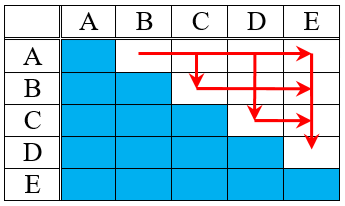The five bale weights will be A, B, C, D, and E, in increasing order.We only need to add each pair in one order, and don’t need to add any bale to itself, so the blue cells will not be used. That leaves ten places to put our ten sums.

Each row or column in the addition table will also be in increasing order, as indicated by the arrows.

We start, as always, with the lowest and highest two sums, which the arrows clearly say are in the first two cells of the first row and the last two cells of the last column: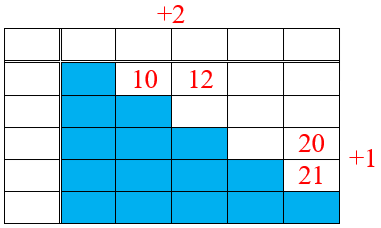(Red indicates what’s new.) I have placed those four numbers, and noted that the second and third bales must differ by 2, and the third and fourth must differ by 1, as indicated by the sums we’ve filled in.

Row and column headings (bale weights) are the same, so what is true of rows is also true of columns; that lets us fill in another row difference and another column difference, and therefore fill in two more sums.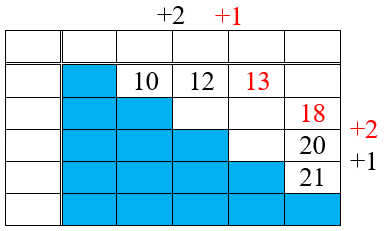Now we have 14, 15, 16, and 17 left to fill in. The smallest, 14, can go in two places. If it were in the upper right, then the last two columns would differ by 1, and we would need a sum of 19 next to the 20. Therefore, the 14 must go below the 12, and we can fill in the rest: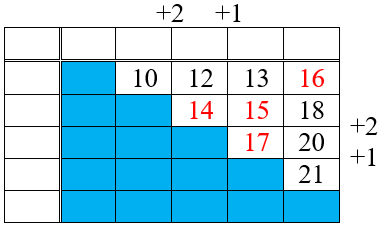Now we can fill in the remaining differences: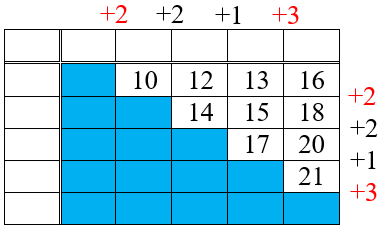All that’s left is to find the actual weights! We see that 10 is the sum of two numbers that differ by 2, so they must be 4 and 6; from there we can fill in the rest: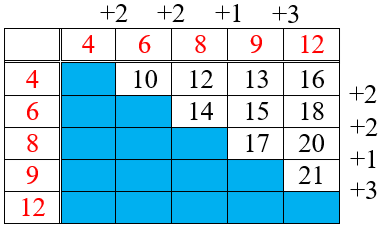Finally, we add 35 back on to each of these numbers, and we have the answer: 39, 41, 43, 44, and 47.

Certainly this method was a lot longer than the quick algebra technique. But it teaches logical reasoning, and required no algebra.

This site uses Akismet to reduce spam. Learn how your comment data is processed.